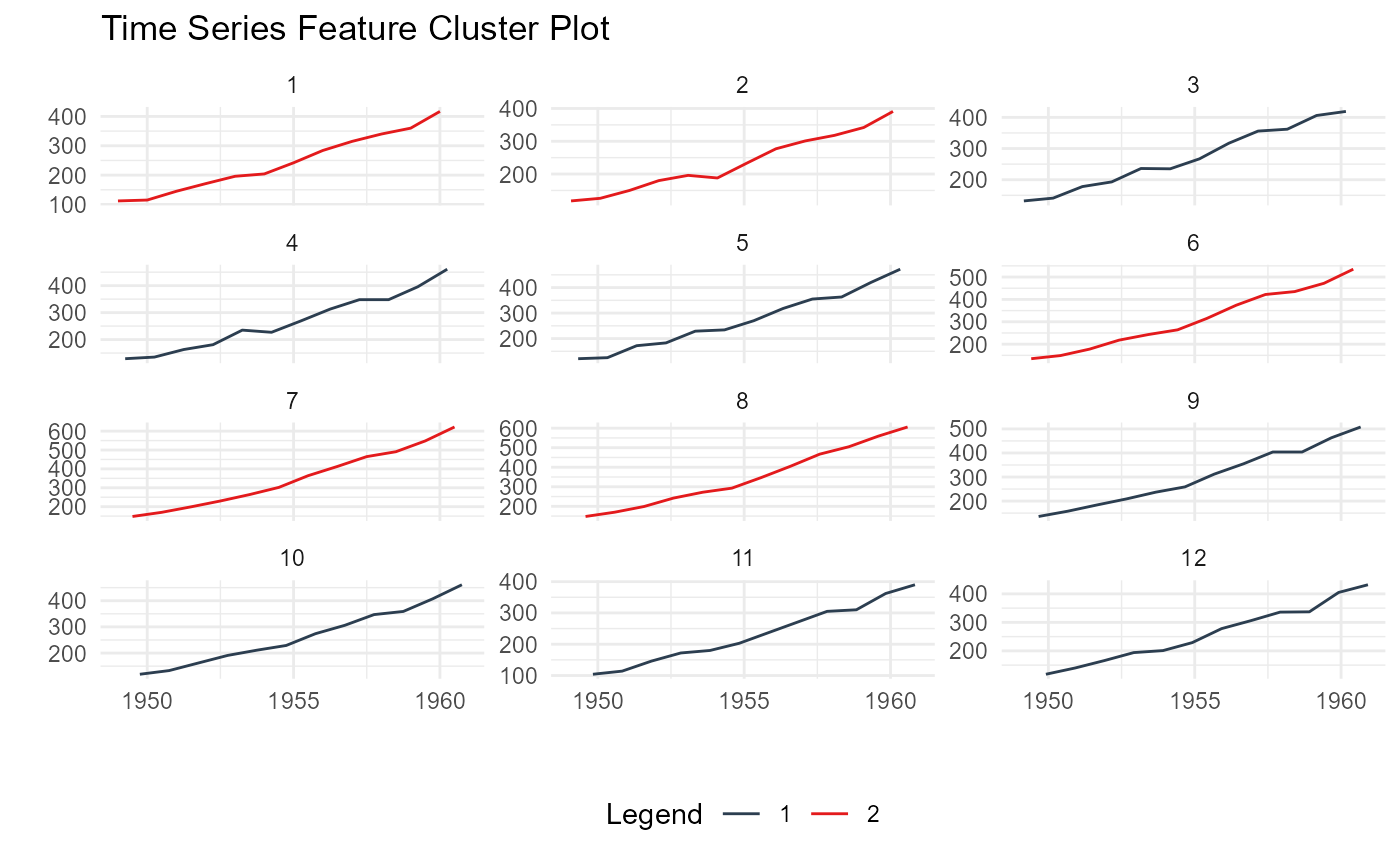This function returns an output list of data and plots that come from using the K-Means clustering algorithm on a time series data.

## Usage

ts_feature_cluster_plot(
.data,
.date_col,
.value_col,
...,
.center = 3,
.facet_ncol = 3,
.smooth = FALSE
)

## Arguments

.data

The data passed must be the output of the ts_feature_cluster() function.

.date_col

The date column.

.value_col

The column that holds the value of the time series that the featurs were built from.

...

This is where you can place grouping variables that are passed off to dplyr::group_by()

.center

An integer of the chosen amount of centers from the ts_feature_cluster() function.

.facet_ncol

This is passed to the timetk::plot_time_series() function.

.smooth

This is passed to the timetk::plot_time_series() function and is set to a default of FALSE.

A list output

## Details

This function will return a list object output. The function itself requires that the ts_feature_cluster() be passed to it as it will look for a specific attribute internally.

The output of this function includes the following:

Data Section

• original_data

• kmm_data_tbl

• user_item_tbl

• cluster_tbl

Plots

• static_plot

• plotly_plot

K-Means Object

• k-means object

Other Clustering: ts_feature_cluster()

## Author

Steven P. Sanderson II, MPH

## Examples


library(dplyr)

data_tbl <- ts_to_tbl(AirPassengers) %>%
mutate(group_id = rep(1:12, 12))

output <- ts_feature_cluster(
.data = data_tbl,
.date_col = date_col,
.value_col = value,
group_id,
.features = c("acf_features","entropy"),
.scale = TRUE,
.prefix = "ts_",
.centers = 3
)

ts_feature_cluster_plot(
.data = output,
.date_col = date_col,
.value_col = value,
.center = 2,
group_id
)
#> Joining, by = "group_id"